CBSE Class 10 Sample Paper for 2019 Boards

Class 10
Solutions of Sample Papers for Class 10 Boards

Question 16 (OR 1 st question)

The points A(1, –2) , B(2, 3), C (k, 2) and D(–4, –3) are the vertices of a parallelogram. Find the value of k.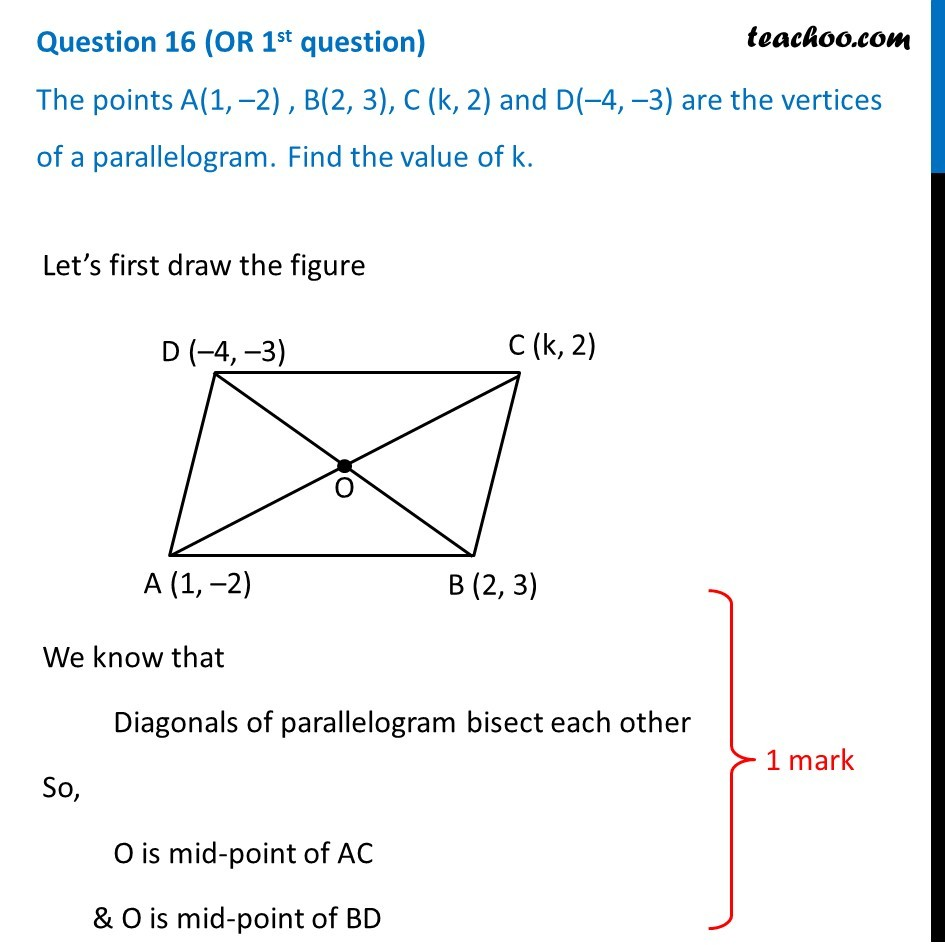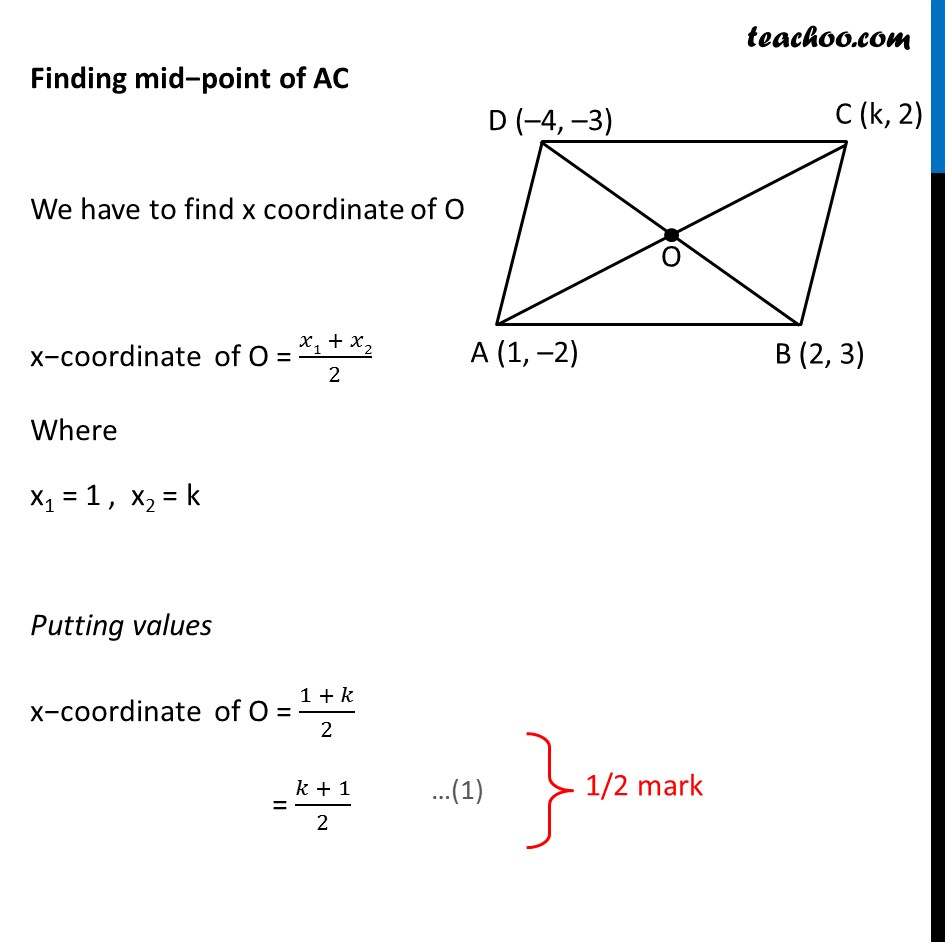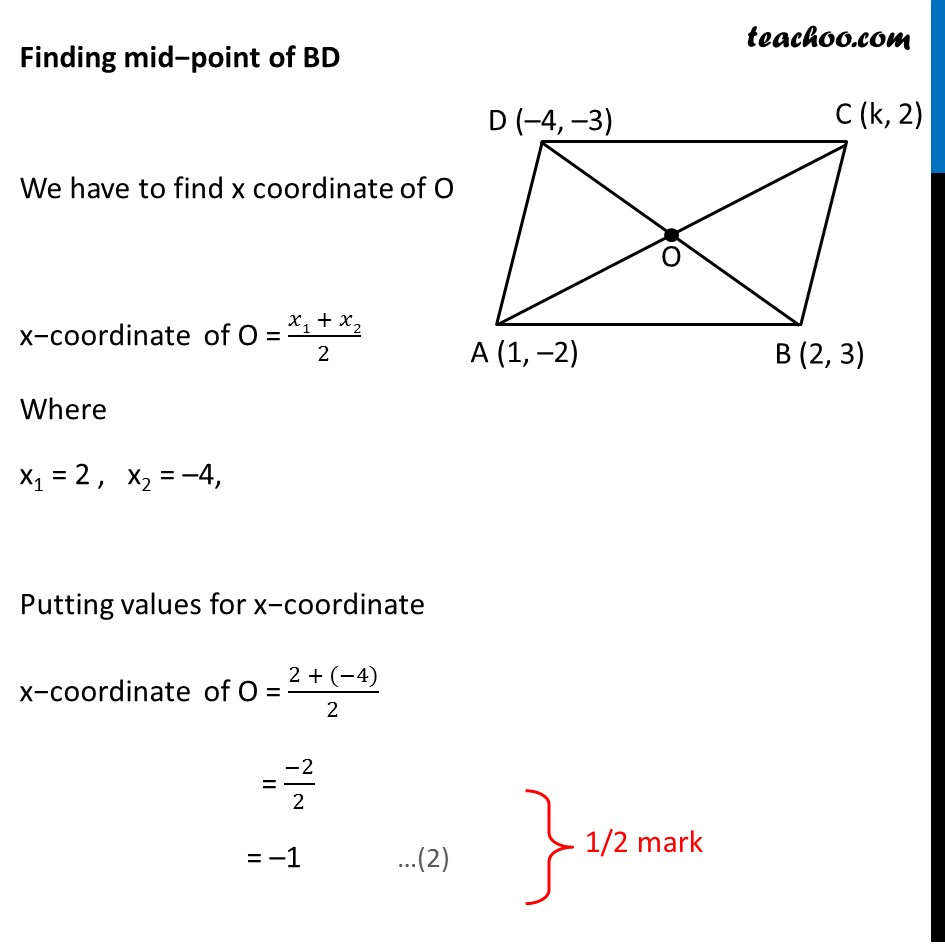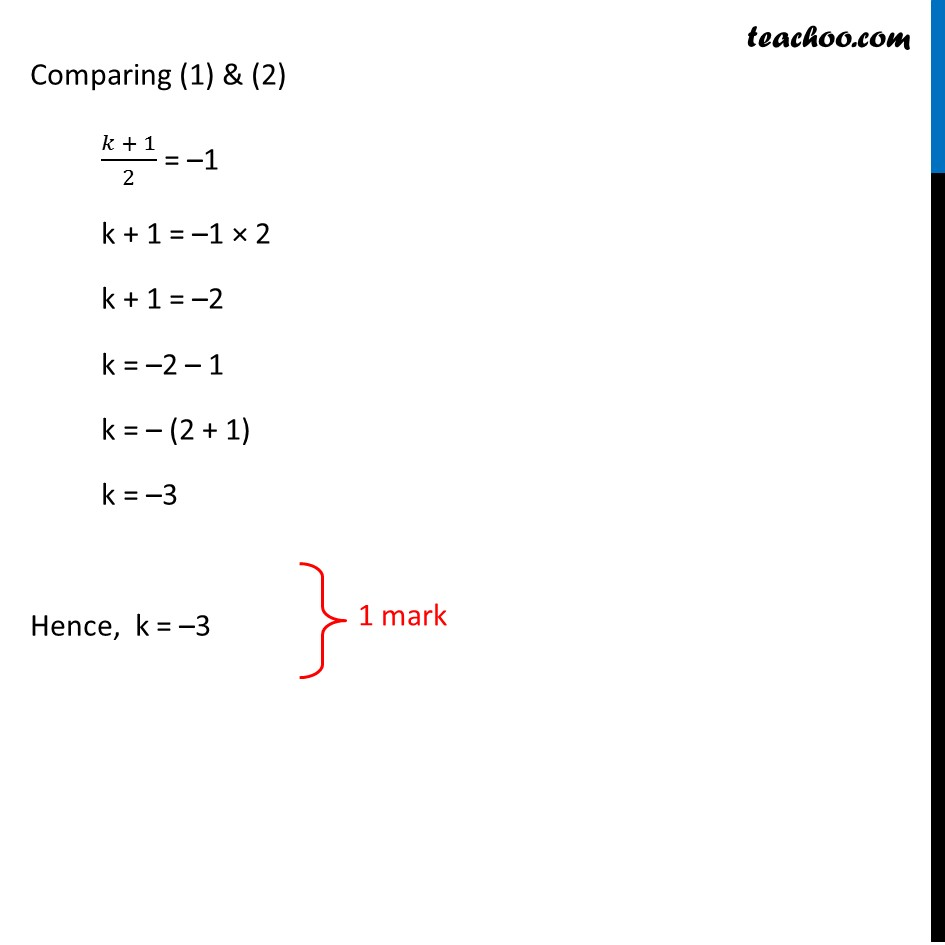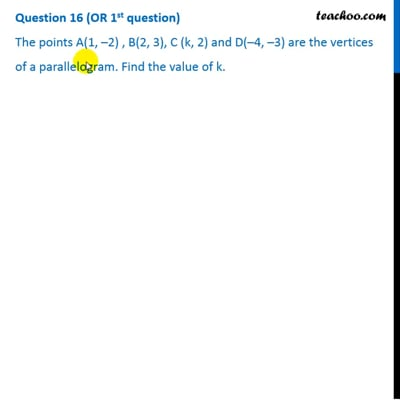This video is only available for Teachoo black users

### Transcript

Question 16 (OR 1st question) The points A(1, –2) , B(2, 3), C (k, 2) and D(–4, –3) are the vertices of a parallelogram. Find the value of k. Let’s first draw the figure We know that Diagonals of parallelogram bisect each other So, O is mid-point of AC & O is mid-point of BD 1 mark Finding mid−point of AC We have to find x coordinate of O x−coordinate of O = (𝑥1 + 𝑥2)/2 Where x1 = 1 , x2 = k Putting values x−coordinate of O = (1 + 𝑘)/2 = (𝑘 + 1)/2 1/2 mark Finding mid−point of BD We have to find x coordinate of O x−coordinate of O = (𝑥1 + 𝑥2)/2 Where x1 = 2 , x2 = –4, Putting values for x−coordinate x−coordinate of O = (2 + (−4))/2 = (−2)/2 = –1 …(2) 1/2 mark Comparing (1) & (2) (𝑘 + 1)/2 = –1 k + 1 = –1 × 2 k + 1 = –2 k = –2 – 1 k = – (2 + 1) k = –3 Hence, k = –3 1 mark